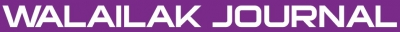### Evaluation of Gas Solid Reactions in Packed Bed Applying Differential Transformation Method

Arash YAHYAZADEH, Hossein YAHYAZADEH, Behnam KHOSHANDAM, Mofid Gorji BANDPY

#### Abstract

Differential transformation method (DTM), followed by Laplace transformation, has been used to solve the governing mass balance equations through a packed bed of solid reactants. The principle of this method is briefly introduced and is then applied to the nonlinear mass balance equations. Conversion and dimensionless gas concentration has been achieved and plotted with different values of Dz. Results show that conversion increases with a high rate initially, and the rate of increasing will decrease dramatically in infinity. All obtained results have been compared with the experimental results, which represent the high accuracy of the presented method. DTM is an efficient analytical technique which is used to solve the nonlinear differential equations, governing the problem in the form of series with easily computable terms.

doi:10.14456/WJST.2014.62

#### Keywords

Gas solid reactions, packed bed, differential transformation method (DTM), Laplace transform, analytical methods

PDF

#### References

GF Froment and KB Bischoff. Chemical Reactor Analysis and Design. John Wiley & Sons, New York, 1979.

BS Sampath, PA Ramachandran and R Hughes. Modeling of non-catalytic gas solid reactions-II. Transient simulation of a packed-bed reactor. Chem. Eng. Sci. 1975; 30, 135-43.

MA Hastaoglu and BE Jibril. Transient modeling of hydrodesulphurization in a fixed-bed reactor. Chem. Eng. Community 2003; 190, 151-70.

P Gupta and RK Saha. Mathematical modeling of non-catalytic fluid-solid reactions Generalized mathematical modeling of fluid-solid non-catalytic reactions using finite volume method: Isothermal analysis. J. Chem. Eng. Jpn. 2003; 36, 1308-17.

P Gupta and RK Saha. Mathematical modeling of non-catalytic fluid-solid reactions Generalized mathematical modeling of fluid-solid non-catalytic reactions using finite volume method: Non-isothermal analysis. J. Chem. Eng. Jpn. 2003; 36, 1298-307.

MS Valipour and Y Saboohi. Modeling of non-catalytic gas-solid reactions in a moving bed of porous pellets based on finite volume method. Heat Mass Tran. 2007; 43, 881-94.

BA Finlayson. Orthogonal collocation on finite elements. Chem. Eng. Sci. 1975; 30, 587-96.

ABM Heesink, W Prins and WPV Swaaij. A grain size distribution model for non-catalytic gas solid reactions. Chem. Eng. J. 1993; 53, 25-37.

JK Zhou. Differential Transform and Its Application for Electrical Circuits. Huarjung University Press, Wuuhahn, China, 1986.

MJ Jang, CL Chen and YC Liu. Analysis of the response of a strongly nonlinear damped system using a differential transformation technique. Appl. Math. Comput. 1997; 88, 137-51.

CL Chen, YC Liu and J Optim. Solution of two-boundary-value problems using the differential transformation method. J. Optim. Theor. Appl. 1998; 99, 23-35.

MJ Jang, CL Chen and YC Liu. On solving the initial-value problems using the differential transformation method. Appl. Math. Comput. 2000; 115, 145-60.

S Momani and Z Odibat. Generalized differential transform method for solving a space- and time-fractional diffusion-wave equation. Phys. Lett. A 2007; 370, 379-87.

Z Odibat and S Momani. Approximate solutions for boundary value problems of time-fractional wave equation. Appl. Math. Comput. 2006; 181, 1351-8.

Z Odibat and S Momani. Numerical methods for nonlinear partial differential equations of fractional order. Appl. Math. Model. 2008; 32, 28-39.

BL Kuo. Heat transfer analysis for the FalknereSkan wedge flow by the differential transformation method. Int. J. Heat Mass Tran. 2005; 48, 5036-46.

BL Kuo. Application of the differential transformation method to the solutions of the free convection problem. Appl. Math. Comput. 2005; 165, 63-79.

Y Keskin, A Kurnaz, ME Kiris and G Oturanc. Approximate solutions of generalized pantograph equations by the differential transform method. Int. J. Nonlinear Sci. Numer. Simulat. 2007; 8, 159-64.

Y Keskin and G Oturanc. The reduced differential transform method: a new approach to fractional partial differential equations. Nonlinear Sci. Lett. A 2010; 1, 207-17.

JS Chiou and JR Tzeng. Application of the Taylor transform to nonlinear vibration problems. J. Vib. Acoust. 1996; 118, 83-7.

MJ Jang, CL Chen and YC Liu. Two-dimensional differential transform for partial differential equations. Appl. Math. Comput. 2001; 121, 261-70.

IH Abdel-Halim Hassan. On solving some eigenvalue-problems by using a differential transformation. Appl. Math. Comput. 2002; 127, 1-22.

AA Joneidi, DD Ganji and M Babaelahi. Differential transformation method to determine fin efficiency of convective straight fins with temperature dependent thermal conductivity. Int. Comm. Heat Mass Tran. 2009; 36, 757-62.

ZZ Ganji, DD Ganji and Y Rostamiyan. Solitary wave solutions for a time-fraction generalized Hirota-Satsuma coupled KdV equation by an analytical technique. Appl. Math. Model. 2009; 33, 3107-13.

M Jalaal and DD Ganji. An analytical study on motion of a sphere rolling down an inclined plane submerged in a Newtonian fluid. Powder Tech. 2010; 198, 82-92.

ZZ Ganji, DD Ganji and H Bararnia. Approximate general and explicit solutions of nonlinear BBMB equations by Exp-Function method. Appl. Math. Model. 2009; 33, 1836-41.

DD Ganji, A Sadighi and I Khatami. Assessment of two analytical approaches in some nonlinear problems arising in engineering sciences. Phys. Lett. A 2008; 372, 4399-406.

DD Ganji and A Sadighi. Application of He’s homotopy-perturbation method to nonlinear coupled systems of reaction-diffusion equations. Int. J. Nonlinear Sci. Numer. Simulat. 2006; 7, 411-8.

DD Ganji and A Rajabi. Assessment of homotopy perturbation and perturbation methods in heat transfer radiation equations. Int. Comm. Heat Mass Tran. 2006; 33, 391-400.

M Gorji, DD Ganji and S Soleimani. New application of He's homotopy perturbation method. Int. J. Nonlinear Sci. Numer. Simulat. 2007; 8, 319-28.

DD Ganji, M Rafei, A Sadighi and ZZ Ganji. A comparative comparison of He's method with perturbation and numerical methods for nonlinear vibrations equations. Int. J. Nonlinear Dyn. Eng. Sci. 2009; 1, 1-20.

CK Chen and SP Ju. Application of differential transformation to transient advective-dispersive transport equation. Appl. Math. Comput. 2004; 155, 25-38.

CK Chen and SS Chen. Application of the differential transformation method to a nonlinear conservative system. Appl. Math. Comput. 2004; 154, 431-41.

MG Ranade and JW Evans. The reaction between a gas and a solid in a non-isothermal packed bed reactor: Simulation and experiments. Ind. Eng. Chem. Process Des. Dev. 1980; 19, 118-23.

### Refbacks

• There are currently no refbacks.Online ISSN: 2228-835X

http://wjst.wu.ac.th

Last updated: 12 August 2019